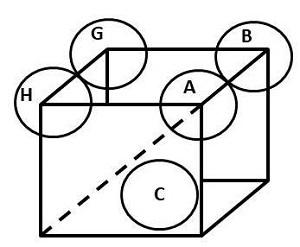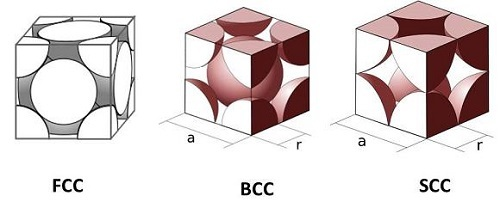# Unit Cell Packing Efficiency

## Introduction

Unit cell of packing efficiency is referred to the development of the particles of constituents of any specific structure or cell with the presence of void spaces between the atoms. The matter of packing cells is obtained with any specific volume that occupied by the particles that are constituent with the total volume of cells. Packing of the unit cell efficiency is often described with a percentage value.

## Information regarding Unit Cell Packing Efficiency

A unit cell is described as a structure having three dimensions and constructed by one or more atoms. Additionally, the 3D object is used to make to view a unit cell. The moment when there is packing in the unit cell, it has been found that a certain void is available in it.

The stretch is loaded by other components or particles. The trace of absolute space that is replenished with the special cell or structure is named the packing fraction. In Chemistry a percentage view is essential for the development of knowledge regarding the effectiveness of the particles that are constituents. The notion of the structure delivered effective knowledge regarding the unit cell packing in chemistry.

A lattice referred to a largely constructed number of unit cells in which the point of the lattice is either filled or occupied by a constituent particle. The point of lattice for a unit cell is a concept that seeks a structure of three-dimensional that is referred by a 3D structure also.

The cubic closed or packed or the cap and the hexagonal closed packed or hcp are two efficient lattices with the consideration of packing in chemistry. Both of the packing refer to the 74% of the space is filled. For instance, a cubic lattice has a packing efficiency of 52.4% and 68% for the body-centred cubic lattice or bcc.

## Packing Efficiency of the Body–Centred Cubic (BCC) Unit Cell

BCC is the kind of atomic arrangement array refers to the particles available on eight edges of a box structure and a single particle at the centre of body or box. The following formula is taken into consideration for the efficiency with the process of calculation based on the following expression −

$$\mathrm{Radius\:or\:r =\sqrt{\frac{3}{4}}\:a}$$

Additionally, the above-furnished equation keeps two atoms and area occupied by one atom is $\mathrm{\frac{4}{3}}$. The efficiency of BCC Packing is considered as 68.04% and rest of 32% of the volume remains void.

## Packing Efficiency of Simple Cubic Unit CellFigure 1: Packing Efficiency in Body-centred Cubic Unit Cell

$$\mathrm{r=\frac{a}{2}}$$

$$\mathrm{a=2r}$$

Thus, packing efficiency = volume occupied by one atom total volume of unit cell x 100.

$$\mathrm{Packing\:Efficiency = \frac{4}{3}r^3 (2r^3 \times\:100)}$$

Hence, the packing efficiency of the simple cubic cell (SCC) unit is 52.4%.

## Packing Efficiency of Hexagonal Close Packing (HCP) and Cubic Close Packing (CCP)

Both the HCP and CCP are referred to the structure that has identical packing efficiency with the relation between both sides is presented as the radius are presented as r.Figure 2: Efficiency of FCC, BCC, and simple packing of atoms

Cubique_centre_atomes_par_maille.svg: Cdang (original idea and SVG execution), Samuel Dupré (3D modelling with SolidWorks) derivative work: Daniele Pugliesi (talk), CCC crystal cell (opaque), CC BY-SA 3.0

Cdang, Cubique a faces centrees atomes par maille, CC BY-SA 3.0

CCC_crystal_cell_(opaque).svg: *Cubique_centre_atomes_par_maille.svg: Cdang (original idea and SVG execution), Samuel Dupré (3D modelling with SolidWorks) derivative work: Daniele Pugliesi (talk) derivative work: Daniele Pugliesi (talk), Simple cubic crystal cell (opaque), CC BY-SA 3.0

The following equation referred to the use case of both packing technique in solutions −

$$\mathrm{a = \sqrt{2r}}$$

These blocks referred to as the face-centred lattice point of the cubic and it contains some extra atoms where these are situated on eight corners of the cube and the centre of each wall as well. The volume represents with r and the equation can be stated as the following matter −

$$\mathrm{(2\sqrt{2}r)^3 = 16\sqrt{3}{2r}^3}$$

The HCP and CCP are referred to as 26% of the void space in the efficiency of packing.

## Conclusion

The unit cell is referred to the smallest collection of molecules or atoms forms a crystal when these are referred to as regularly intervals in 3D. The proportion of the total void area to the occupied position by atoms or ions referred to as the packing efficiency in the unit cell. The Hexagonal close packaging is referred to the cubic structures developed by organising and focusing on the body.

## FAQs

Q1. What is packing efficiency?

Ans. The percentage of the overall space, which is occupied by the particles in a certain packing, is comprehended as packing efficiency. Atoms, ions, and other particles that constitute are packed closely in the crystal lattice. These can do the process inside the ccp or in the hcp based on the phenomenon. Both of the cases referred to the free space in the number where the void is left, the total space is not occupied.

Q2. What is the packing efficiency in HCP and CCP structure?

Ans. The efficiency of the packing in the CCP and FCC is a cubic structure entered for the face. The atoms in the octahedral void are referred to as the cubic close packing (ccp) is 74%. Additionally, two different names are there in comprehending knowledge of packing efficiency.

Q3. Is CCP and FCC the same?

Ans. The CCP and FCC are the cubic stress that is referred to the development of knowledge regarding the packing of the entered atoms in the cell unit. The void of the packing refers to the ABCABC form that is known as CCP and the unit cell is FCC.

Q4. What is the process of BCC packing in the unit cell?

Ans. The addition of the BCC packing in the chemistry provides knowledge regarding the process of eight atoms in each corner and one atom placed in the centre. The structure of BCC is open in the efficiency of unit cell packing. The equation refers to 8 × 1/8 = 1 atom.

Q5. What is the packing efficiency in SCC?

Ans. The efficiency rate of packing of SSC referred to around 52.4% in the unit cell packing in chemistry.

Updated on: 17-Apr-2023

500 Views